# Fractional Forms of Repeating Decimals

A system of equations can be used to determine the fractional form of a repeating decimal.

For instance, to find the fractional form of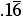, let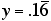.Then multiplying both sides by 10 results in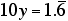.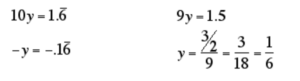What are the simple fractions for the following repeating decimals?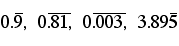How would you convert any repeating decimal to a simple fraction?
Explain all possible cases.